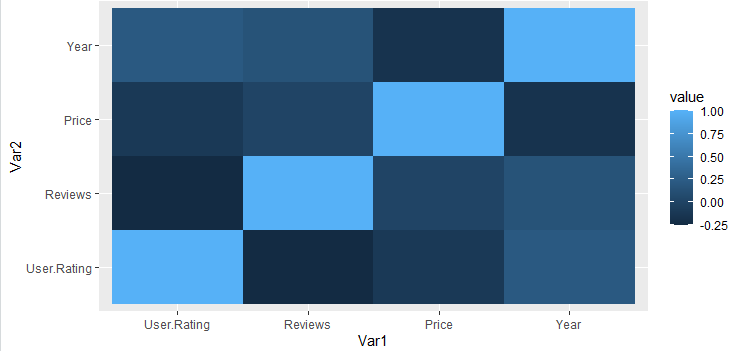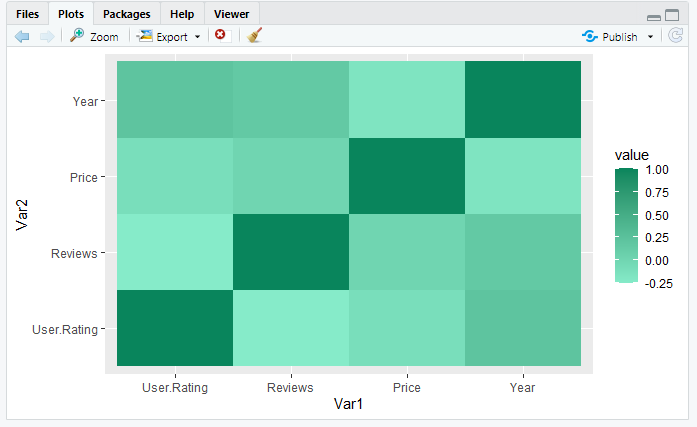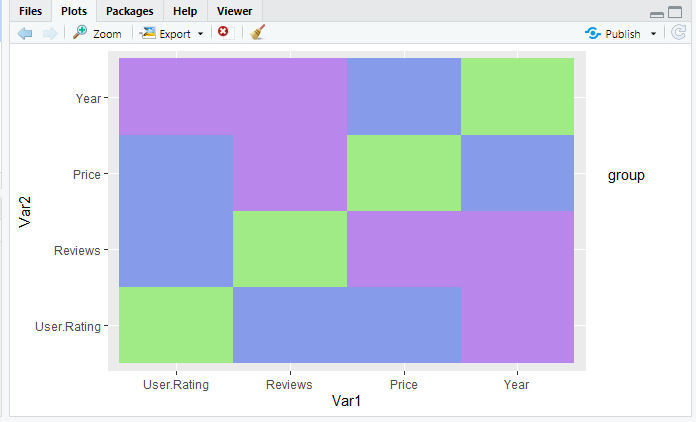Related Articles

# Change Color of Range in ggplot2 Heatmap in R

• Last Updated : 18 Jul, 2021

A heatmap depicts the relationship between two attributes of a dataframe as a color-coded tile. A heatmap produces a grid with multiple attributes of the dataframe, representing the relationship between the two attributes taken at a time.

Dataset in use: bestsellers

Let us first create a regular heatmap with colors provided by default. We will use geom_tile() function of ggplot2 library. It is essentially used to create heatmaps.

Syntax: geom_tile(x,y,fill)

Parameter:

• x: position on x-axis
• y: position on y-axis
• fill: numeric values that will be translated to colors

To this function, Var1 and Var2 of the melted dataframe are passed to x and y respectively. These represent the relationship between attributes taken two at a time. To fill parameters provide, since that will be used to color-code the tiles based on some numeric value.

Example:

## R

 `library``(ggplot2)``library``(reshape2)`` ` `df<-``read.csv``(``"bestsellers.csv"``)`` ` `data<-``cor``(df[``sapply``(df,is.numeric)])``data1<-``melt``(data)`` ` `ggplot``(data1,``       ``aes``(x=Var1,``           ``y=Var2,``           ``fill=value))+``geom_tile``()`

Output:In this method, the starting and the ending value of the colors to define a range is given as an argument.

Parameter:

• low: starting value
• high: ending value
• guide: type of legend

Example:

## R

 `library``(ggplot2)``library``(reshape2)`` ` `df<-``read.csv``(``"bestsellers.csv"``)``data<-``cor``(df[``sapply``(df,is.numeric)])``data1<-``melt``(data)`` ` `ggplot``(data1,``aes``(x=Var1,``                 ``y=Var2,``                 ``fill=value))+``geom_tile``()+``scale_fill_gradient``(low = ``"#86ebc9"``,``                    ``high = ``"#09855c"``,``                    ``guide = ``"colorbar"``)`

Output:### Method 2: Using scale_fill_manual()

Up until now, we were adding colors to the continuous values, in this method, the values are first converted into discrete ranges using cut() function.

Syntax: cut(data, breaks)

Where breaks take a vector with values to divide the data by. Now again plot a heatmap but with the new data created after making it discrete. To add colors to such heatmap in ranges, use scale_fill_manual() with a vector of the colors for each range.

Syntax: scale_fill_manual(interval, values=vector of colors)

Example:

## R

 `library``(ggplot2)``library``(reshape2)`` ` `df<-``read.csv``(``"bestsellers.csv"``)``data<-``cor``(df[``sapply``(df,is.numeric)])``data1<-``melt``(data)`` ` `data2<-data1``data2\$group<-``cut``(data2\$value,``                 ``breaks = ``c``(-1,-0.5,0,0.5, 1))`` ` ` ` `ggplot``(data2,``aes``(x=Var1,``                 ``y=Var2,``                 ``fill=group))+``geom_tile``()+``  ``scale_fill_manual``(breaks = ``levels``(data2\$group),``                    ``values = ``c``(``"#86ebc9"``, ``"#869ceb"``,``                               ``"#b986eb"``,``"#a1eb86"``))`

Output:My Personal Notes arrow_drop_up